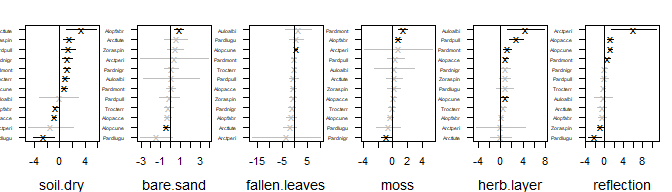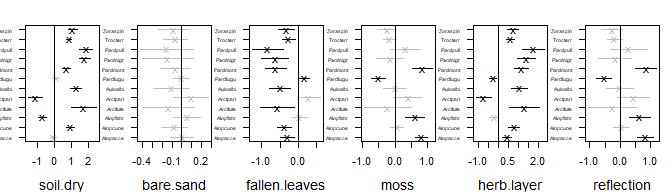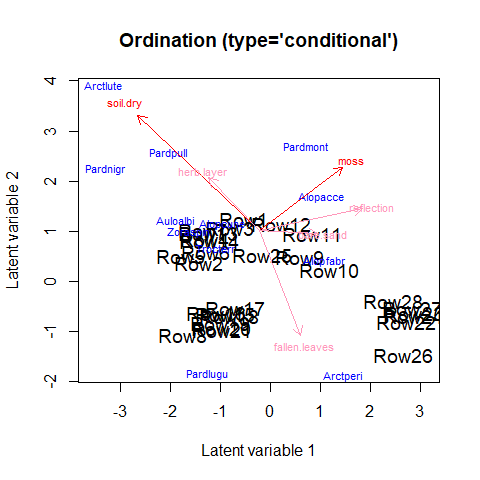# Ordination with predictors

#### 2023-09-18

Until recently, the gllvm R-package only supported unconstrained ordination. When including predictor variables, the interpretation of the ordination would shift to a residual ordination, conditional on the predictors.

However, if the number of predictor variables is large and so is the number of species, including predictors can result in a very large number of parameters to estimate. For data of ecological communities, which can be quite sparse, this is not always a reasonable model to fit. As alternative, ecologists have performed constrained ordination for decades, with methods such as Canonical Correspondence Analysis, or Redundancy Analysis. Alternatively, these methods can be viewed as determining “gradients” (a.k.a. latent variables) as a linear combination of the predictor variables.

In this vignette, we demonstrate how to include predictors directly in an ordination with the gllvm R-package. Methods are explained with details in van der Veen et al. (2022). We start by loading the hunting spider dataset:

library(gllvm)

data(spider)
Y <- spider$abund X <- spider$x

#And scale the predictors
X <- scale(X)

which includes six predictor variables: “soil.dry”: soil dry mass, “bare.sand”: cover bare sand, “fallen.leaves”: “cover of fallen leaves”, “moss”: cover moss, “herb.layer”: cover of the herb layer, “reflection”: “reflection of the soil surface with a cloudless sky”.

## Constrained ordination

Let us first consider what constrained ordination actually is. We will do that by first shortly explaining reduced rank regression (RRR). First, consider the model:

$\eta_{ij} = \beta_{0j} + \boldsymbol{X}_i^\top\boldsymbol{\beta}_j.$

Here, $$\boldsymbol{\beta}_j$$ are the slopes that represent a species responses to $$p$$ predictor variables at site $$i$$, $$\boldsymbol{X}_i$$. In the gllvm R-package, the code to fit this model is:

MGLM <- gllvm(Y, X = X, family = "poisson", num.lv = 0)

where we set the number of unconstrained latent variables to zero, as it defaults to two. The “rank” of $$\boldsymbol{X}_i^\top\boldsymbol{\beta}_j$$ is $$p$$. Constrained ordination introduces a constraint on the species slopes matrix, namely on the number of independent columns in $$\boldsymbol{\beta}_j$$ (a column is not independent when it can be formulated as a linear combination of another). The reduced ranks are in community ecology referred to as ecological gradients, but can also be understood as ordination axes or latent variables. If we define a latent variable $$\boldsymbol{z}_i = \boldsymbol{B}^\top\boldsymbol{X}_{i,lv} + \boldsymbol{\epsilon}_i$$, for a $$p\times d$$ matrix of slopes, we can understand constrained ordination as a regression of the latent variable or ecological gradient, except that the residual $$\boldsymbol{\epsilon}_i$$ is omitted, i.e. we assume that the ecological gradient can be represented perfectly by the predictor variables, so that the model becomes: $\begin{equation} \eta_{ij} = \beta_{0j} + \boldsymbol{X}_i^\top\boldsymbol{B}\boldsymbol{\gamma}_j. \end{equation}$

Where $$\boldsymbol{B}$$ is a $$d \times K$$ matrix of slopes per predictor and latent variable, and $$\boldsymbol{\gamma}_j$$ is a set of slopes for each species per latent variable. This parametrization is practically useful, as it drastically reduces the number of parameters compared to multivariate regression. The rank, number of latent variables or ordination axes, can be determined by cross-validation, or alternatively by using information criteria. The code for this in the gllvm R-package, for an arbitrary choice of two latent variables, is:

RRGLM <- gllvm(Y, X = X, family = "poisson", num.RR = 2)

The predictor slopes (called canonical coefficients in e.g., CCA or RDA) are available under RRGLM$params$LvXcoef or can be retrieved with coef(RRGLM) or by summary(RRGLM):

summary(RRGLM, rotate = TRUE)
##
## Call:
## gllvm(y = Y, X = X, family = "poisson", num.RR = 6, starting.val = "zero",
##     reltol.c = 1e-15)
##
## Family:  poisson
##
## AIC:  1841.216 AICc:  1898.108 BIC:  2161.853 LL:  -836.6 df:  84
##
## Informed LVs:  0
## Constrained LVs:  6
## Unconstrained LVs:  0
##
## Formula:  ~ 1
## LV formula:  ~soil.dry + bare.sand + fallen.leaves + moss + herb.layer + reflection
##
## Coefficients LV predictors:
##                      Estimate Std. Error z value Pr(>|z|)
## soil.dry(CLV1)       0.739241   0.545770   1.354  0.17558
## bare.sand(CLV1)     -1.504227   0.738137  -2.038  0.04156 *
## fallen.leaves(CLV1)  0.249424   1.567803   0.159  0.87360
## moss(CLV1)          -0.406028   1.325428  -0.306  0.75935
## herb.layer(CLV1)     1.983275   1.046501   1.895  0.05807 .
## reflection(CLV1)    -2.069525   0.941468  -2.198  0.02794 *
## soil.dry(CLV2)      -0.524884   0.325749  -1.611  0.10711
## bare.sand(CLV2)     -0.761665   0.395216  -1.927  0.05395 .
## fallen.leaves(CLV2) -0.877375   0.969414  -0.905  0.36543
## moss(CLV2)           0.130217   0.659360   0.197  0.84344
## herb.layer(CLV2)     1.793405   0.584467   3.068  0.00215 **
## reflection(CLV2)     0.315377   0.600692   0.525  0.59957
## soil.dry(CLV3)       0.750252   0.988362   0.759  0.44780
## bare.sand(CLV3)     -0.897121   0.928729  -0.966  0.33406
## fallen.leaves(CLV3) -1.145885   1.649139  -0.695  0.48716
## moss(CLV3)          -0.342914   0.768733  -0.446  0.65554
## herb.layer(CLV3)    -1.345636   1.047277  -1.285  0.19883
## reflection(CLV3)     0.820609   0.674645   1.216  0.22385
## soil.dry(CLV4)      -0.997668   0.514773  -1.938  0.05261 .
## bare.sand(CLV4)     -0.522736   0.432766  -1.208  0.22709
## fallen.leaves(CLV4) -0.606122   1.004898  -0.603  0.54640
## moss(CLV4)           0.150650   0.698787   0.216  0.82931
## herb.layer(CLV4)     0.026434   0.669088   0.040  0.96849
## reflection(CLV4)    -1.211241   0.496380  -2.440  0.01468 *
## soil.dry(CLV5)       0.592508   0.230516   2.570  0.01016 *
## bare.sand(CLV5)      0.430542   0.196589   2.190  0.02852 *
## fallen.leaves(CLV5) -0.814613   0.341699  -2.384  0.01713 *
## moss(CLV5)           0.289127   0.182575   1.584  0.11328
## herb.layer(CLV5)    -0.160839   0.253159  -0.635  0.52521
## reflection(CLV5)    -0.726094   0.149143  -4.868 1.12e-06 ***
## soil.dry(CLV6)      -0.031456   0.020354  -1.545  0.12224
## bare.sand(CLV6)      0.004485   0.014587   0.307  0.75850
## fallen.leaves(CLV6) -0.057097   0.033731  -1.693  0.09051 .
## moss(CLV6)          -0.086429   0.020041  -4.313 1.61e-05 ***
## herb.layer(CLV6)    -0.015565   0.019434  -0.801  0.42316
## reflection(CLV6)    -0.009270   0.014017  -0.661  0.50840
## ---
## Signif. codes:  0 '***' 0.001 '**' 0.01 '*' 0.05 '.' 0.1 ' ' 1

Note the use of the rotate argument. This ensures that the solution of the canonical coefficients is rotated to the same solution as displayed in ordiplot (which has the same rotate argument that is by default set to TRUE). The summary can be useful in determining what drives vertical and horizontal shifts in the location of species and sites in the ordination diagram (but probably not for much more than that).

Unlike the canonical coefficients, the reconstructed species-specific effects are invariant to rotation, so that it is always good practice to additionally study those for more exhaustive inference;

coefplot(RRGLM, cex.ylab = 0.5, mar = c(4,2,2,1))To fit a constrained ordination we make use of additional optimization routines from the and R-packages. This is necessary, because without the constraint that the predictor slopes are orthogonal the model is unidentifiable. It might thus happen, that after fitting the model a warning pops-up along the lines of predictor slopes are not orthogonal, in which case you will have to re-fit the model with a different optimization routine (optimizer='alabama') or starting values (starting.values='zero') in order to get the constraints on the canonical coefficients to better converge.

Note: in general to improve convergence, it is good practice to center and scale the predictor variables.

### Random slopes

Generally, constrained ordination can have difficulty in estimating the predictor slopes, for example due to co-linearity between the predictors. One way to solve this, is using regularisation. Regularisation adds a penalty to the objective function, as to shrink parameter estimates closer to zero that are unimportant. A convenient way to add this penalty, is by formulating a random slopes model. In gllvm this is done with the following syntax:

RRGLMb1 <- gllvm(Y, X = X, family="poisson", num.RR = 2, randomB = "LV")
RRGLMb1 <- gllvm(Y, X = X, family="poisson", num.RR = 2, randomB = "P")

where the randomB argument is additionally used to specify if variances of the random slopes should be unique per latent variable (i.e. assume that the random slopes per predictor come from the same distribution), or per predictor (i.e. assume that the random slopes per latent variable come from the same distribution). Either setting has benefits, the first implies covariance between species responses to a predictor, whereas the latter can serve to shrink effects of a single predictor to near zero. In general, it has the potential to stabilize model fitting and reduce variance of the parameter estimates. Finally, a slope for a categorical predictor is an intercept, so this formulation also allows to include random intercepts in the ordination.

A plot of species-specific effects can be constructed with the randomCoefplot function:

randomCoefplot(RRGLMb1, cex.ylab = 0.5, mar = c(4,2,2,1))When using a random-effects formulation, there is no summary table and there are no confidence intervals available for the canonical coefficients. It is possible to construct a summary table with the prediction of the random-effects and the association prediction errors as follows:

# Get coefficients
coefs<-data.frame(coef(RRGLMb1,"LvXcoef"))
coefs$Predictor <- row.names(coefs) # Wide to long coefsTab<-reshape(coefs, direction = "long", varying = list(colnames(coefs)[1:(RRGLMb1$num.lv.c+RRGLMb1$num.RR)]), v.names = "Estimate", times=colnames(coefs)[1:(RRGLMb1$num.lv.c+RRGLMb1$num.RR)], timevar = "LV", new.row.names = NULL) # Add prediction errors coefsTab <- cbind(coefsTab,PE=c(getPredictErr(RRGLMb1)$b.lv))
row.names(coefsTab)<-1:nrow(coefsTab)
coefsTab[,-4]
##        Predictor   LV     Estimate         PE
## 1       soil.dry CLV1 -0.080723064 0.07814185
## 2      bare.sand CLV1  0.001068039 0.06094532
## 3  fallen.leaves CLV1 -0.297420665 0.10174450
## 4           moss CLV1  0.809784152 0.09236949
## 5     herb.layer CLV1  0.447862820 0.10908394
## 6     reflection CLV1  0.836470551 0.13821746
## 7       soil.dry CLV2  0.945275422 0.12950352
## 8      bare.sand CLV2 -0.075514139 0.04851537
## 9  fallen.leaves CLV2 -0.212377414 0.23493650
## 10          moss CLV2 -0.386609004 0.64863215
## 11    herb.layer CLV2  0.547191839 0.37130075
## 12    reflection CLV2 -0.413646154 0.69906377

There is a range of potential use cases for random canonical coefficients, but pragmatically, inducing some shrinkage on the predictor effects can result in more better “behaved” (fewer outliers) ordination plots.

## Concurrent ordination

Unlike in other R-packages, we can now formulate a ordination where additional random-effects are included that act like LV-level residuals (because let’s face it, how often are we 100% confident that we have measured all relevant predictors?), so that we can assume that the ecological gradient is represented by unmeasured and measured predictors (the former is how the residual can be understood). The code for this is:

CGLLVM <- gllvm(Y, X = X, family = "poisson", num.lv.c = 2)

where the num.lv.c argument is used to specify the number of latent variables for the concurrent ordination (i.e. latent variables informed by the predictors but not constrained), where previously the num.RR argument was used to specify the number of constrained latent variables. The number of constrained, informed, and unconstrained latent variables can be freely combined using the num.RR, num.lv.c and num.lv arguments (but be careful not to overparameterize or overfit your model!). It is also possible to combine those arguments with full-rank predictor effects. To combine concurrent ordination with full-rank predictor effects you need to use the formula interface:

PCGLLVM <- gllvm(Y, X = X, family = "poisson", num.lv.c = 2,
lv.formula = ~bare.sand + fallen.leaves + moss+herb.layer + reflection,
formula = ~soil.dry)

where lv.formula is the formula for the ordination with predictors (concurrent or constrained), and X.formula is the formula which tells the model which predictors should be modelled in full-rank. Note, that those two formulas cannot include the same predictor variables, and all predictor variables should be provided in the X argument. In essence, this performs a partial concurrent ordination. Constrained ordination should not include an (additional) intercept as it can be re-parameterized into a model with only $$\beta_{0j}$$.

Though we did not do so here, information criteria can be used to determine the correct number of latent variables. Results of the model can be examined in more details using the summary(\cdot) function:

summary(CGLLVM)
##
## Call:
## gllvm(y = Y, X = X, num.lv.c = 2, family = "poisson", starting.val = "zero")
##
## Family:  poisson
##
## AIC:  1665.321 AICc:  1680.282 BIC:  1840.908 LL:  -786.7 df:  46
##
## Informed LVs:  2
## Constrained LVs:  0
## Unconstrained LVs:  0
## Residual standard deviation of LVs:  0.6577 0.5729
##
## Formula:  ~ 1
## LV formula:  ~soil.dry + bare.sand + fallen.leaves + moss + herb.layer + reflection
##
## Coefficients LV predictors:
##                     Estimate Std. Error z value Pr(>|z|)
## soil.dry(CLV1)      -0.98389    0.33529  -2.934 0.003342 **
## bare.sand(CLV1)      0.47349    0.23214   2.040 0.041380 *
## fallen.leaves(CLV1)  0.33758    0.39012   0.865 0.386866
## moss(CLV1)           0.67915    0.31526   2.154 0.031224 *
## herb.layer(CLV1)    -0.40845    0.22679  -1.801 0.071706 .
## reflection(CLV1)     0.83825    0.37495   2.236 0.025376 *
## soil.dry(CLV2)       0.93546    0.26428   3.540 0.000401 ***
## bare.sand(CLV2)     -0.02443    0.19079  -0.128 0.898113
## fallen.leaves(CLV2) -0.84909    0.31374  -2.706 0.006803 **
## moss(CLV2)           0.51290    0.23897   2.146 0.031851 *
## herb.layer(CLV2)     0.42712    0.19684   2.170 0.030013 *
## reflection(CLV2)     0.17366    0.31602   0.550 0.582646
## ---
## Signif. codes:  0 '***' 0.001 '**' 0.01 '*' 0.05 '.' 0.1 ' ' 1

Finally, we can use all the other tools in the R-package for inference, such as creating an ordination diagram with arrows:

ordiplot(CGLLVM, biplot = TRUE)Arrows that show as less intense red (pink), are predictors of which the confidence interval for the slope includes zero, for at least one of the two plotted dimensions. There are various arguments included in the function to improve readability of the figure, have a look at its documentation. The arrows are always proportional to the size of the plot, so that the predictor with the largest slope estimate is the largest arrow. If the predictors have no effect, the slopes $$\boldsymbol{B}$$ will be close to zero.

It is also possible to use the quadratic flag to fit a quadratic response model though we will not demonstrate that here, or to partition variance per latent variable and for specific predictors.